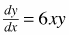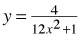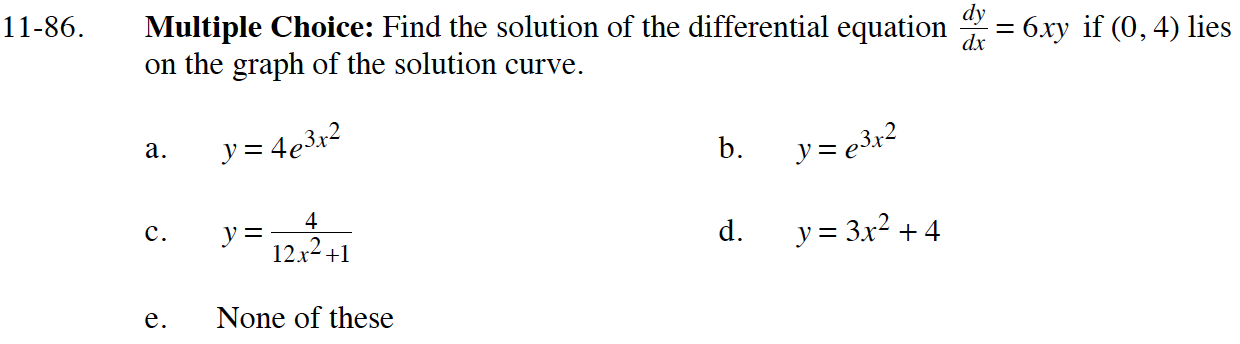### Home > CALC > Chapter 11 > Lesson 11.2.4 > Problem11-86

11-86.
1. Multiple Choice: Find the solution of the differential equationif (0, 4) lies on the graph of the solution curve. Homework Help ✎

1. y = 4e3x 2

2. y = e3x 2

3.4. y = 3x2 + 4

5. None of theseUse the point (0, 4) to solve for the value of C.